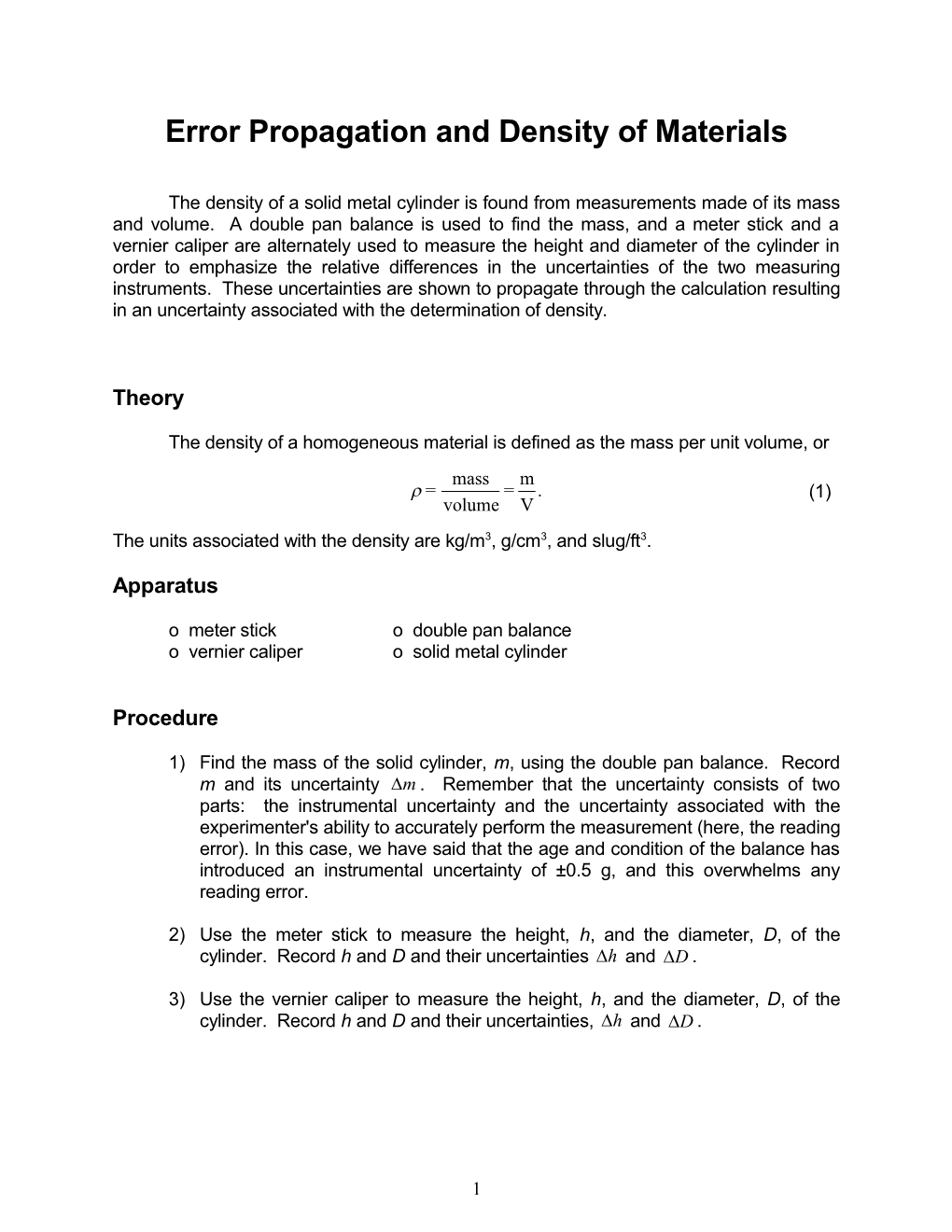# Error Propagation and Density of Materials## Error Propagation and Density of Materials

The density of a solid metal cylinder is found from measurements made of its mass and volume. A double pan balance is used to find the mass, and a meter stick and a vernier caliper are alternately used to measure the height and diameter of the cylinder in order to emphasize the relative differences in the uncertainties of the two measuring instruments. These uncertainties are shown to propagate through the calculation resulting in an uncertainty associated with the determination of density.

Theory

The density of a homogeneous material is defined as the mass per unit volume, or

1(1)

The units associated with the density are kg/m3, g/cm3, and slug/ft3.

Apparatus

o meter sticko double pan balance

o vernier calipero solid metal cylinder

Procedure

1)Find the mass of the solid cylinder, m, using the double pan balance. Record m and its uncertainty . Remember that the uncertainty consists of two parts: the instrumental uncertainty and the uncertainty associated with the experimenter's ability to accurately perform the measurement (here, the reading error). In this case, we have said that the age and condition of the balance has introduced an instrumental uncertainty of ±0.5 g, and this overwhelms any reading error.

2)Use the meter stick to measure the height, h, and the diameter, D, of the cylinder. Record h and D and their uncertainties and .

3)Use the vernier caliper to measure the height, h, and the diameter, D, of the cylinder. Record h and D and their uncertainties, and .

Analysis

The density of a sample of material is given by (1), that is,

2(1)

where m is the mass of the sample and V is the volume. The volume of a cylinder is

3(7)

where D is the diameter and h is the height of the cylinder. The density can then be written as

4(8)

and the uncertainty in the density as

5(9)

Use (8) and (9) and the meter stick measurements to calculate the density of the sample and its uncertainty.

Use (8) and (9) and the vernier caliper measurements to calculate the density and its uncertainty.

Discussion

Compare your two experimental values of density with the true densities of metals and decide which metal you actually had. Plot on a one-dimensional graph the experimental results with their error ranges, labeled “ruler” and “vernier caliper”. Plot the true value of the density on the same graph. Label this value as the "best known value."

State which instrument gave the most precise value and which gave the most accurate value, and an explanation of your choices. Did the two measuring instruments perform as you expected? What was the percentage difference between the true value and each of the experimental values?

Conclusion

State the two experimental densities and their uncertainties and what metal you had.